## MP Board Class 8th Maths Solutions Chapter 2 Linear Equations in One Variable Ex 2.1

Solve the following equations.

Question 1.
x – 2 = 7.
Solution:
We have, x – 2 = 7
Transposing -2 to R.H.S., we get
x = 7 + 2 ⇒ x = 9, which is the required solution.

Question 2.
y + 3 = 10.
Solution:
We have, y + 3 = 10
Transposing 3 to R.H.S., we get y = 10 – 3
⇒ y = 7, which is the required solution.

Question 3.
6 = z + 2.
Solution:
We have, 6 = z + 2
Transposing 2 to L.H.S., we get
6 – 2 = z
⇒ z = 4, which is the required solution.Question 4.
$$\frac{3}{7}$$ + x = $$\frac{17}{7}$$.
Solution: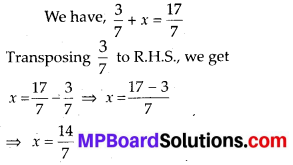⇒ x = 2, which is the required solution.

Question 5.
6x = 12.
Solution:
We have, 6x = 12
Dividing both sides by 6, we get
$$\frac{6 x}{6}=\frac{12}{6}$$
⇒ x = 2, which is the required solution.Question 6.
$$\frac{t}{5}=10$$
Solution:
We have, $$\frac{t}{5}$$ = 10
Multiplying both sides by 5, we get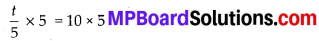⇒ t = 50, which is the required solution.

Question 7.
$$\frac{2 x}{3}$$ = 18
Solution:
We have,
$$\frac{2 x}{3}$$ = 18
Dividing both sides by 2, we get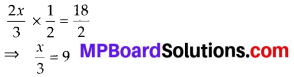Now, multiplying both sides by 3, we get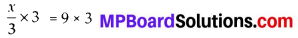⇒ x = 27, which is the required solution.Question 8.
$$1.6=\frac{y}{1.5}$$
Solution:
We have, $$1.6=\frac{y}{1.5}$$
Multiplying both sides by 1.5, we get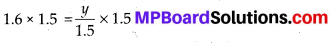⇒ y = 2.4, which is the required solution.Question 9.
7x – 9 = 16.
Solution:
We have, 7x – 9 = 16
Transposing -9 to R.H.S., we get
7x = 16 + 9
⇒ 7x = 25
Now, dividing both sides by 7, we get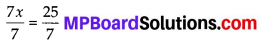⇒ x = $$\frac{25}{7}$$, which is the required solution.Question 10.
14y – 8 = 13.
Solution:
We have, 14y -8 = 13
Transposing -8 to R.H.S., we get
14y = 13 + 8 ⇒ 14y = 21
Now, dividing both sides by 14, we get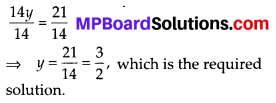Question 11.
17 + 6p = 9.
Solution:
We have, 17 + 6p = 9
Transposing 17 to R.H.S., we get 6p = 9 – 17
⇒ 6p = – 8
Now, dividing both sides by 6, we get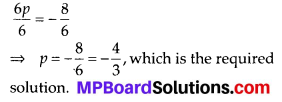Question 12.
$$\frac{x}{3}+1=\frac{7}{15}$$
Solution: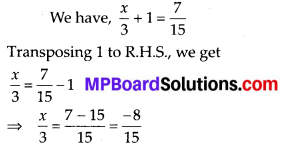Now, multiplying both sides by 3, we get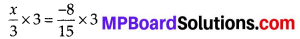⇒ x = $$-\frac{8}{5}$$, which is the required solution.